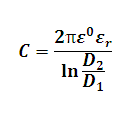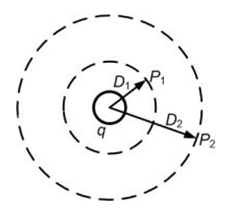# Capacitance of Straight Conductor Calculator

This Calctown Calculator calculates the capacitance of a straight conductor.

Here e0 = 8.854 * 10-12. Please enter er accordingly.

unit
m
m

#### Result

FClick here to view image

where

ε0 = Dielectric constant in vaccum

εr = Dielectric ratio

D1, D2 are distances from conductor

C = Capacitance of conductor

q = charge on conductor